# How to Convert a Decimal to a Fraction

How to Convert a Decimal to a Fraction• To write a decimal as a fraction we write the digits above the place value column that the last digit is in.
• The last digit of 0.93 is the 3 which is in the hundredths column.
• Here is 93 hundredths so it is written as 93 out of 100,  93 / 100  .
• The number of decimal places in the number tells us how many zeros will be on the denominator of the fraction.
• 0.93 has 2 decimal places and so it is out of 100, which is written with 2 zeros.
A decimal number is written as a fraction by writing the digits after the decimal point above the place value column that its last digit is in.• In the decimal number 2.607, we have a whole number part of 2 and a decimal part of .607.
• The whole number part can be written separately, so we write the 2 down first.
• The last digit of the decimal part is the 7, which is in the thousandths column.
• We write the decimal digits out of 1000.
• 2.607 is written as a fraction as 2   607 / 1000  .Supporting Lessons

#### Converting a Decimal to a Mixed Number# Writing Decimal Numbers as Fractions

## How to Convert a Decimal to a Fraction

To convert a decimal to a fraction follow these steps:

1. Write the decimal digits as the numerator of the fraction.
2. Write the place value column of the last decimal digit as the denominator of the fraction.
3. Simplify the fraction if necessary.

The decimal place value columns from left to right are the tenths, hundredths and thousandths.The last digit of the decimal number determines what the denominator on the bottom of the fraction is.For example, the last digit of 0.9 is in the tenths column and so the fraction will be out of 10.The only digit of the decimal number is 9 and so, 9 is the numerator of the fraction.

The decimal number 0.9 is written as a fraction as   9 / 10  .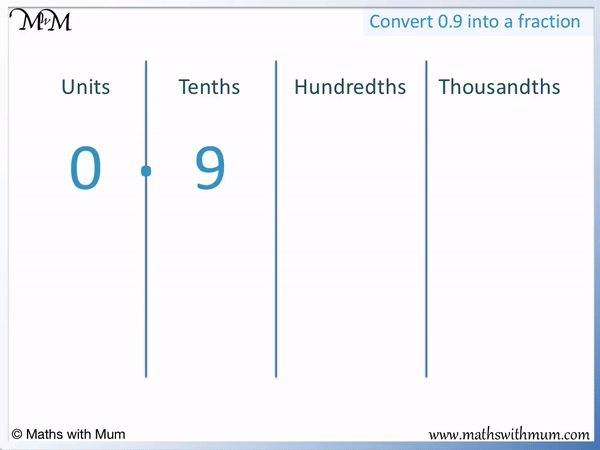If there is only one decimal place in the decimal number, the fraction is written out of 10.

Here is an example of writing a decimal number in the hundredths as a fraction.The last digit of 0.93 is in the hundredths column and so, the fraction is out of 100.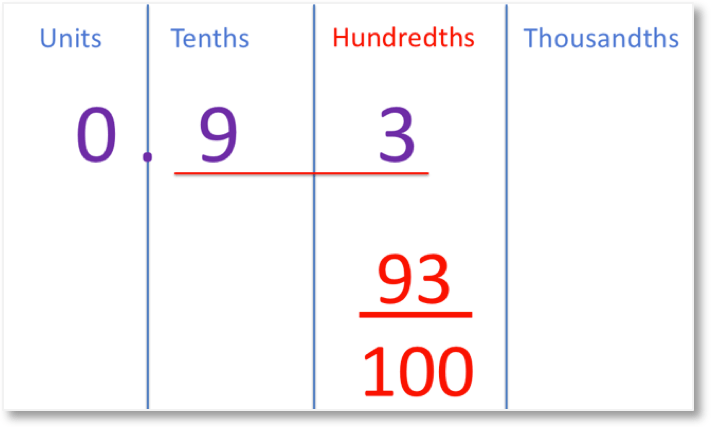The decimal digits are 93, so the fraction is   93 / 100  .If there are two decimal places in the decimal number, the fraction is written out of 100.

Here is an example of writing a decimal number in the thousandths as a fraction.The last digit of 0.239 is in the thousandths column, so the fraction is out of 1000.We write the decimal digits 239 as the numerator.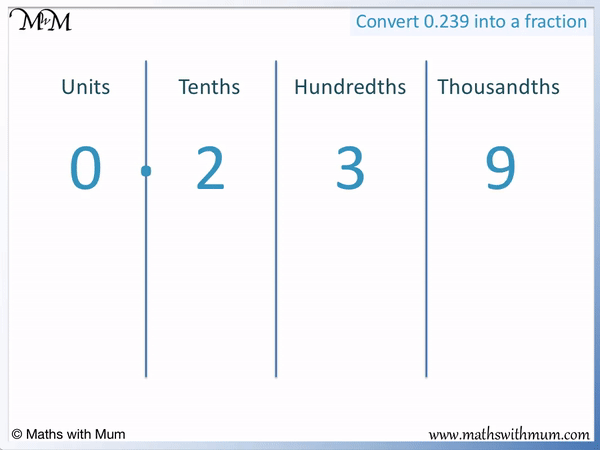The decimal number 0.239 as a fraction is   239 / 1000  .

If there are three decimal places in the decimal number, the fraction is written out of 1000.

Here is another example of writing the decimal 0.043 as a fraction.The last digit of 0.043 is in the thousandths column, so the fraction is out of 1000.

We only look at the digits after the first zero and so, the decimal digits of 43 go as the numerator.

0.043 is written as a fraction as   43 / 1000  .

Here is the decimal number 0.101.

The last digit of 0.101 is in the thousandths column so the fraction is written out of 1000.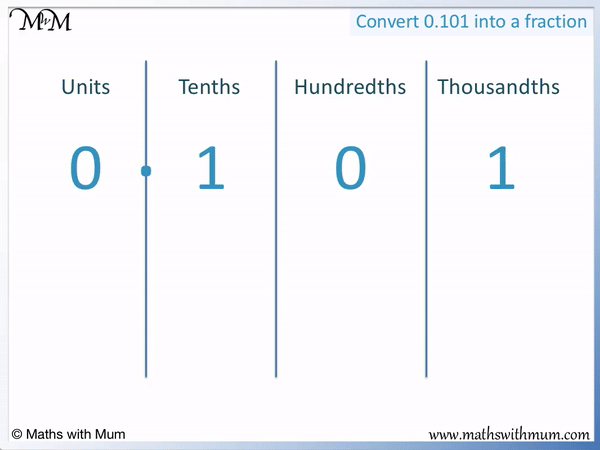The decimal digits are 101. We write 0.101 as a fraction as   101 / 1000  .

## Converting Decimals to Mixed Numbers

To convert a decimal to a mixed number follow these steps:

1. Write the digits before the decimal point down as the whole number part of the answer.
2. Write the digits after the decimal point as the numerator in the fraction of the answer.
3. Write the place value column of the last decimal digit as the denominator.

A mixed number is a combination of a whole number and a fraction written alongside another.

When the decimal number is larger than 1, it will convert into a mixed number.

For example, here is the decimal number of 5.33.The first step is to write the digit before the decimal point as the whole number part of the answer. We write 5 down as the whole number part of the answer.

Next we form the fraction by looking at the decimal digits.

The last digit is in the hundredths column and so, the fraction is out of 100.

The decimal digits after the decimal point are 33, so we write 33 out of 100.

The decimal 5.33 written as a mixed number is 5   33 / 100  .

Here is another example of writing a decimal as a mixed fraction. We have 7.03.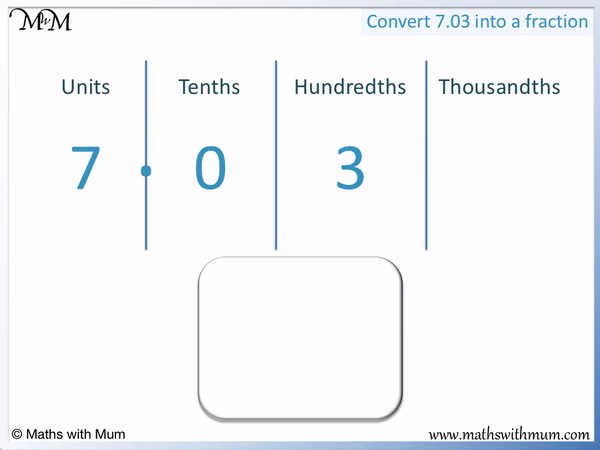We first write the 7 as the whole number part of the mixed number.

The last decimal digit is in the hundredths column and so, the fraction is out of 100.

The first non-zero digit after the decimal point is 3 and so, the numerator is 3.7.03 is written as a fraction as 7   3 / 100  .

Here is an example of writing the decimal 2.607 as a mixed number.We first write the whole number 2.

The last digit is in the thousandths column and so the fraction is out of 1000.

The numerator is 607.2.607 written as a mixed number is 2   607 / 1000  .

## How to Write a Decimal as a Fraction in Simplest Form

To write a decimal as a fraction in simplest form, write the decimal digits above the place value column of the last decimal digit. To simplify, divide the numerator and denominator by a number that divides exactly into both of them.

For example, here is the decimal number 3.2. We will write it as a fraction in simplest form.

We first write the whole number part of 3 separately.The last digit of 3.2 is in the tenths column and so, the fraction is out of 10.

We put the decimal digit of 2 as the numerator.

3.2 is written as a fraction as 3   2 / 10  .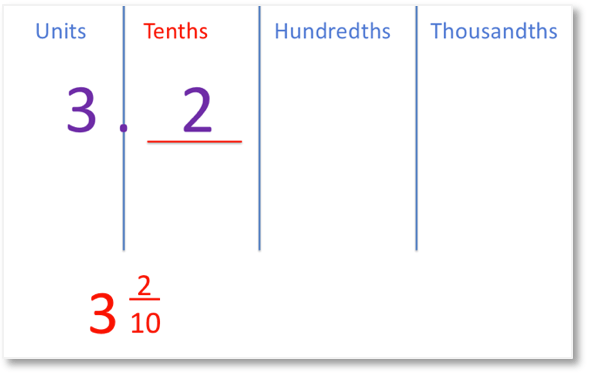However, both 2 and 10 are even and can be divided by 2.

Dividing 2 by 2 gives us a numerator of 1 and then dividing 10 by 2 gives us a denominator of 5.

We simplify the fraction   2 / 10   to   1 / 5  .

Therefore the decimal number of 3.2 can be written as a simplified fraction as 3   1 / 5  .Now try our lesson on Negative Numbers on a Number Line where we learn about negative numbers.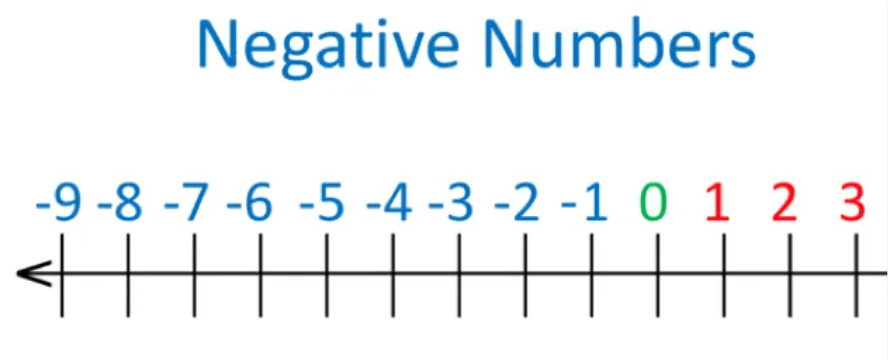error: Content is protected !!